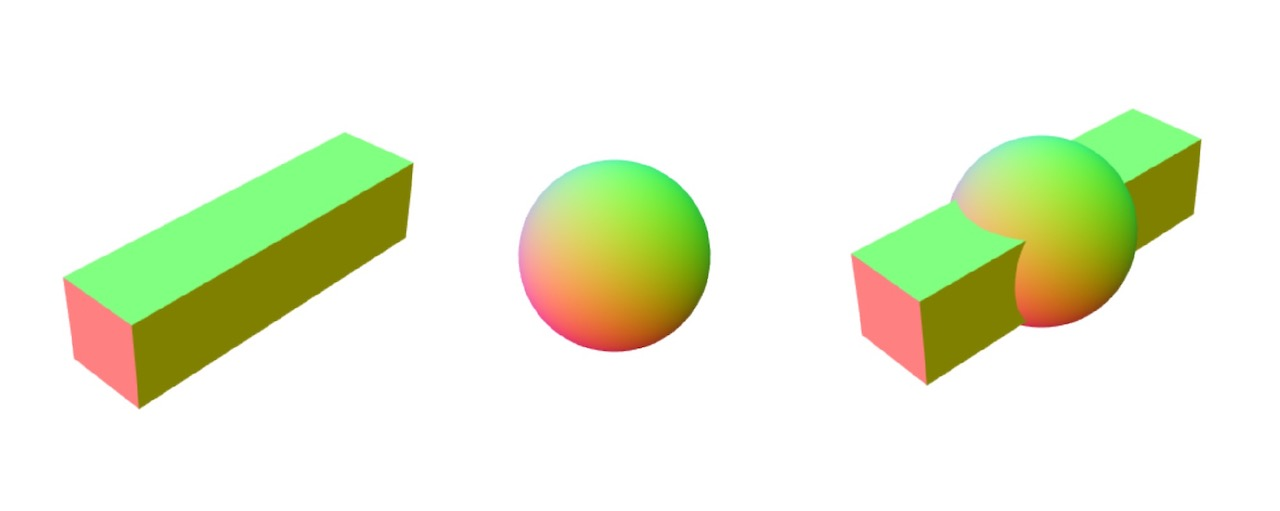# geom-mergeMerges multiple geometries into one.

## Usage

``````var merge = require('geom-merge')
var cube = require('primitive-cube')()
var sphere = require('primitive-sphere')()

var g = merge([cube, sphere])
``````

## API

### `merge(geometries)`

• `geometries` - array of geometry objects

Returns new geometry with merged attributes and cells from provided geometries.

Note 1: Each geometry object requires at least `positions` (array of [x, y, z]) and `cells` (array of [i, j, k]) properties. Other properties like `uvs` or `normals` will be merged as well if available in all geometries. Note 2: This module doesn't perform CSG operations